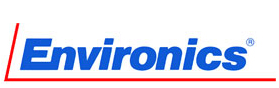# Calculating Mass Flow Conversion Factors

The production of precise calibration gas standards relies heavily on the accurate control of gas flows through devices known as mass flow controllers (MFCs). To guarantee the accuracy of MFCs, it is essential to meticulously assess the gas conversion factors (also known as K-factors or GCF) for each process mass flow.Image Credit: Shutterstock

This article explores the significance of gas conversion factors, and the methodology for determining GCF for process gasses is expounded upon.

## The Importance of Calibration Gas Standards

Precisely formulated gas mixtures with known concentrations of particular target gases are referred to as calibration gas standards.

To guarantee the accuracy of gas concentration measurements, almost all equipment must undergo regular calibration using calibration gas standards. This includes gas and chemical sensors, mass spectrometers, gas chromatographs, handheld detectors, and auto emissions analyzers.

Mass flow controllers (MFCs), devices capable of measuring fluid mass flow rates with exceptional precision and automatically adjusting gas flow using a valve, are employed to prepare calibration gas standards.

MFCs provide a precise measure of gas flow through a tube that is unaffected by variations in temperature and pressure by measuring the mass flow rate of gases rather than the volume flow rate.1

The flow rate is typically determined by thermal techniques that measure the temperature variation as the gas enters and exits a small tube.

However, specific heat capacities for gases are unique, resulting in a difference in the amount of energy associated with a given temperature change for each gas.2 Consequently, MFCs must be calibrated to ensure the accurate measurement of the mass flow rate for a specific process gas.

## Calibrating Mass Flow Controllers Using Mass Flow Gas Conversion Factors

Ideally, the process gas to be analyzed should be utilized for calibrating an MFC; however, this is not always feasible.

In such cases, a substitute gas may be employed, but this necessitates the use of a mass flow conversion factor (K) to ensure the accuracy of MFC flow relative to the process gas.

Essentially, the gas conversion factor denotes the flow rate ratio required for different gases to generate a specific output voltage from an MFC. All gas conversion factors are established relative to nitrogen, which is conventionally assigned a mass flow conversion factor of one.

The precision of any MFC is contingent on the proper determination of mass flow conversion factors for each process gas used in the blending process. Inaccurate mass flow conversion factors lead to imprecise MFCs, which, in turn, result in inaccurate gas mixing and reference standards.

## Calculating Mass Flow Conversion Factors for Your Process Gases

The ideal method for determining mass flow conversion factors involves empirical calculation. However, companies may use a combination of theoretical and empirical approaches depending on the process gas.

The mass flow conversion factor for a specific gas is defined as the ratio of the actual gas flow rate to the equivalent nitrogen flow rate.

Calculating the mass flow conversion factor for a given gas entails dividing the gas flow rate for that gas by the nitrogen flow rate that produces the identical output voltage from the MFC.

When an alternate gas is used to calibrate the mass flow controller, the ratio applied must be the ratio of the actual process gas to the calibration gas.

Obtaining accurate mass flow conversion factors for all process gases is crucial for developing high-precision gas mixing systems to produce calibration gas standards.

As specialists in gas mixing, the technology at Environics is founded on extremely precise control of thermal mass flow controllers, which enables them to offer exceptional gas mixing capabilities that surpass even the most rigorous requirements.

1. Bramford, T. Using Mass Flow Controller-Based Dynamic Gas Blenders to Produce Accurate Calibration Gas Standards. https://www.environics.com/wp-content/uploads/2020/05/bamford-2007.pdf.
2. Kim, S. J. & Jang, S. P. Experimental and numerical analysis of heat transfer phenomena in a sensor tube of a mass ¯ow controller. International Journal of Heat and Mass Transfer 14 (2001).This information has been sourced, reviewed and adapted from materials provided by Environics, Inc.

## Citations

• APA

Environics, Inc.. (2023, March 08). Calculating Mass Flow Conversion Factors. AZoM. Retrieved on June 06, 2023 from https://www.azom.com/article.aspx?ArticleID=22487.

• MLA

Environics, Inc.. "Calculating Mass Flow Conversion Factors". AZoM. 06 June 2023. <https://www.azom.com/article.aspx?ArticleID=22487>.

• Chicago

Environics, Inc.. "Calculating Mass Flow Conversion Factors". AZoM. https://www.azom.com/article.aspx?ArticleID=22487. (accessed June 06, 2023).

• Harvard

Environics, Inc.. 2023. Calculating Mass Flow Conversion Factors. AZoM, viewed 06 June 2023, https://www.azom.com/article.aspx?ArticleID=22487.